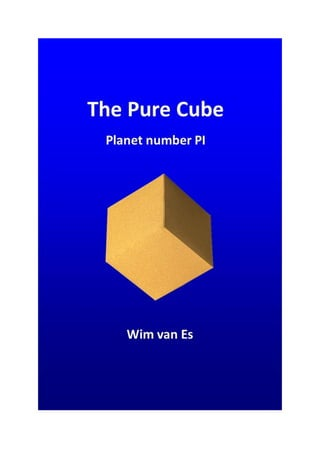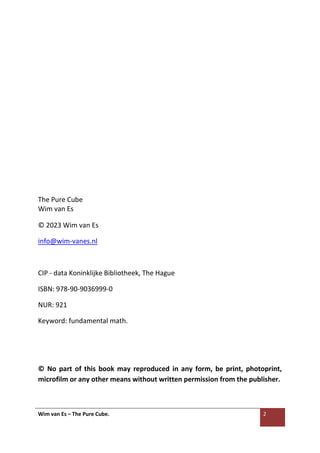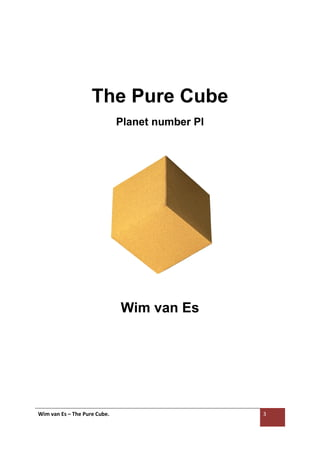Seu SlideShare está sendo baixado. ×

# The Pure Cube.pdf

Anúncio
Anúncio
Anúncio
Anúncio
Anúncio
Anúncio
Anúncio
Anúncio
Anúncio
Anúncio
AnúncioAnúncio
Anúncio
Anúncio
Anúncio
Carregando em…3
×

1 de 22 Anúncio

# The Pure Cube.pdf

This booklet treats the cube in all its facets.
It shows that a cube always contains 4 x the number Pi, in the triangular ratio √1:√2:√3.
The cube shows that planet Earth also has a Pi number.
The cube contains a calendar of the day and year.
My new golden spiral is added once again.

This booklet treats the cube in all its facets.
It shows that a cube always contains 4 x the number Pi, in the triangular ratio √1:√2:√3.
The cube shows that planet Earth also has a Pi number.
The cube contains a calendar of the day and year.
My new golden spiral is added once again.

Anúncio
Anúncio

### The Pure Cube.pdf

1. 1. Wim van Es – The Pure Cube. 2 1. √1 - The Pure Cube Wim van Es © 2023 Wim van Es info@wim-vanes.nl CIP - data Koninklijke Bibliotheek, The Hague ISBN: 978-90-9036999-0 NUR: 921 Keyword: fundamental math. © No part of this book may reproduced in any form, be print, photoprint, microfilm or any other means without written permission from the publisher.
2. 2. Wim van Es – The Pure Cube. 3 The Pure Cube Planet number PI Wim van Es
3. 3. Wim van Es – The Pure Cube. 4 Preface This publication shows how perfect a cube is when you dissect it into all its mathematical facets. I show that the cube is perfect in its shape. That in the cube 5 x the number Pi is hidden. That planet Earth has a unique Pi number. I show that the cube represents a reckoning of time, day and year. This cube is perfect. Wim van Es January 2023
4. 4. Wim van Es – The Pure Cube. 5 Introduction Within Freemasonry one strives as much as possible to become a pure cubic. Freemasons work on their own rough stone to eventually become this pure cubic. The pure cube symbolizes life in the right relationship. This is not always easy. Within Freemasonry, a lot of symbolic language is used within which everyone can perfect their own learning and growth process. We will never be perfect, but the touchstone (cube) keeps us on the right path, if we regularly test our actions against the stone (cube). However, the cube also has a mathematical perfection. And I'm going to describe it now. In the previously published booklets 'Mathematics of the Golden Pyramid' and 'Mathematics of the Great Pyramid' I have already explained the golden triangle and the golden pyramid. It is important to also read these booklets to gain a deeper understanding of certain concepts that I am now going to describe. https://www.slideshare.net/WimvanEs1
5. 5. Wim van Es – The Pure Cube. 6 The cube It is now important to start drawing (designing) the cube in the ratio 6:6:6:6. As you draw the equilateral triangle in the ratio 6:6:6. So these proportions are essential. We are going to draw the cube. Figure 1 Figure 1 What you see is that a cube has 12 equal sides. Each side is 6 cm. The cube has 6 square faces. What is special about this cube that has a mathematical value and a great symbolic value? • The cube contains 4 x the number PI. • In the cube is the planet Earth number PI. • The calculation method is in the cube: diameter – circumference. • The cube contains the time of a day. • The cube contains the number of days in a year. • In the cube, the triangle is in the ratio 1: 2: √5. • There is a timeline in the cube, of seven time phases. We will now start with the first statement.
6. 6. Wim van Es – The Pure Cube. 7 The cube contains 4 x the number PI. If we draw a line from B diagonally to C inside the cube, we get the diagonal size of a square. And that is therefore 6 x √2 = 8,485 … cm. Side A - B = 6 cm = √1. Side A - C is then √1² + √2² = √3² = 36 + 72 = 108. Side A - C = √108 = 10.392 cm. See figure 2. Figure 2 Figure 2 shows the triangle in the ratio √1:√2:√3. You can project it 4 x diagonally within the cube. Figure 3 = 2x. Figure 3
7. 7. Wim van Es – The Pure Cube. 8 If you have now read the books 'Mathematics of the Golden Pyramid' and 'Mathematics of the Great Pyramid' then you know exactly what this unique new triangle means. It is the pyramid and pentagram ratio (√2 + √3) / √1 = PI. 9.43/3 = 3.143333… or 22.64/7.2 = 3.144444… If we are going to calculate the number Pi inside the cube, you get rounded to 2 decimal places: 8.48 + 10.39 = 18.87 cm / 6 = 3.145. Figure 4. This is a unique PI number and I'm going to show you. Figure 4 The cube symbolizes the world.
8. 8. Wim van Es – The Pure Cube. 9 Let's look at previous publications. So, there are so far 4 geometrically proven PI numbers. • The pyramid PI number = 3.143333 …. • The pentagram PI number = 3.144444 …. • The 40 degrees PI number = 3.14269 …. • The square PI number = 3.14269 …. There is no fixed PI number, even though we have assumed this based on ….? At least not on a geometric basis. I am now going to explain the Planet number PI to you. The Earth is a 'circle' and it also has a unique PI number. This unique Planet PI number is exactly 3.14496. I'm going to show you this. I round it in the cube to the PI number 3.145. How are you going to prove this? To begin with, let's take another look at the cube. Figure 5 Figure 5 You can now say that the following calculation is a unique calculation of time, based on this cube.
9. 9. Wim van Es – The Pure Cube. 10 Figure 5 shows that the diagonals of a square face are 8.485 … (6 x √2). Now key in on your calculator: (6 x √2 = …) and that in square. 8.485 …… ² = 72. This 72 is essential in the reckoning of time. If you now multiply 72 by the 12 sides of the cube, you will get an outcome of 864. Now you may ask yourself what I should do with this outcome. Now you're going to multiply 864 by 100, and you'll get a result in seconds of time. This is 86,400 seconds. In case you don't know yet. A day has 86,400 seconds. (24 hours x 3600 seconds). Now the year. Within the current era, there are contradictions that are not in balance with the earlier era. And they don't match our count today either. Let's say a year has 365 days. This is mathematically incorrect. I'm going to show you this. Just a sidetrack. If you read the translation from the book of Enoch (dead sea scrolls), and especially his astronomical articles, you will see that the year has 364 days and not 365. If you check this using an obelisk based on sidereal time (see my previous publications), you will also end up with 364 days. Now let's look at ourselves and hear what they say: a year has 52 weeks, and a week has 7 days. 52 x 7 = 364 days, this is correct.
10. 10. Wim van Es – The Pure Cube. 11 Now the mathematical proof. A day has 86,400 seconds. If we multiply this by 364 days, you get: 86,400 x 364 = 31,449,600 seconds. If we simplify this to 1, you get 31,449,600 / 10,000,000 = 3.14496. This is the perfect Planet Earth number PI = 3.14496. Symbolically captured in this 6 cm cube. So, in the cube we use the rounding 3.145. If we divide the number Pi 3.145 by 0.864, we get 364 days. If we were to calculate all this now with 365 days, you will see that it is not correct. 86,400 x 365 = 31,536,000 = 3,153 and that is not a PI number. The cube thus indicates the day and year in time calculation. It couldn't be more perfect. You can symbolically say that you have the world (and yourself) in your hands. The square plane. If we look closely at the square plane in figure 6, you see the following. Each square has an angle of 54° from the center of one side to the two corners on the opposite side. Figure 6
11. 11. Wim van Es – The Pure Cube. 12 Figure 6 also shows that each square face consists of 4 triangles in the ratio 1:2: √5. The value of this and what you can do with it is explained in the booklets 'Mathematics of the Golden Pyramid' and 'Mathematics of the Great Pyramid'. https://www.slideshare.net/WimvanEs1 So, it's not just a cube. Within the 6 cm cube there is a time reckoning that no one knows. I'm going to explain this one. It comprises seven phases of time, recorded in seven time years. To do this I use all parts of the cube. See figure 7. Figure 7 The cube has an up and down, depending on how you hold it.
12. 12. Wim van Es – The Pure Cube. 13 I'm using the top red square. And all numbers are abstract numbers that I convert into years. There is a multiplier of 100 for it. The top red square area is 4 x 6 = 24 x 100 = 2400. The bottom square blue - green is equal to 4 x 6 = 24 x 100 = 2400. The green part of the bottom square is 3 x 6 = 18 x 100 = 1800. There are 6 squares in total of 24. This is 144. I link this to 12 sides (lines) in the cube that I multiply by 12 = 144. This means that each line is 12 years. 12 x 12 years is 144 years. The remaining four squares (black) represent 100 years each. 4 x 100 = 400 years. We have already seen in figure 5 that the diagonals of a square plane are (6 x √2 = …) and that squared. 8,485 …… ² = 72. This represents 7,200 years. The time calculation I am going to make now is based on seven time phases, 7 time years, in a timeline of 7,200 years.
13. 13. Wim van Es – The Pure Cube. 14 We're going to start with the first step, the first time. This is located at the bottom of the cube. The square area 4 x 6 = 24 x 100 = 2400. I count on the 144 days. Makes 2544. The first time, the first phase is therefore the year 2544 B.C. The second step, the second time, are the three green lines in the bottom square. 3 x 6 = 18 x 100 = 1800. I count down the 144 days. Makes 1656. So, the second time, the second phase is the year 1656 B.C. The third phase, the third step, is the time during which the top and bottom cancel out 2400 – 2400 = 0. And because the year 0 does not exist, the year 1 is meant here. The third time, the third phase is therefore the year 1. Then we go to the fourth step. This is located at the top of the cube. The square 4 x 6 = 24 x 100 = 2400 minus the four squares of 100. (4 x 100 = 400). This makes 2400 – 400 = 2000. The fourth time, the fourth phase is therefore the year 2000. The fifth step, the fifth phase, is the top square itself. 4 x 6 = 24 x 100 = 2400. The fifth time, the fifth phase is therefore the year 2400. The sixth time, the sixth step, is the top square 4 X 6 = 24 x 100 = 2400. I add 3 lines of 12 years = 36. Makes a total of 2436. So, the sixth time, the sixth phase is the year 2436. Then the last, seventh step, the seventh time. The top square 4 x 6 = 24 x 100 = 2400 plus the four squares of 100. (4 x 100 = 400). This makes 2400 + 400 = 2800. The seventh time, the seventh phase is therefore the year 2800.
14. 14. Wim van Es – The Pure Cube. 15 You may wonder what this timeline means? Since this timeline belongs to the 6 cm cube and has a symbolic value if you can interpret it, it completes the cube. This timeline has no exact mathematical value but is based on the cryptic timelines (of the first great light, symbolically speaking) that I have described in the booklet "Secret of the Great Pyramid". They are almost equal and that is why the timeline of the cube can be called unique. The Golden Spiral. We know the Golden spiral of Fibonacci, realized from squares. I am now going to show you a new golden spiral that has been hidden in the Great Pyramid for centuries. It is a spiral from the equilateral triangle. Instead of raising the square in two steps, you are now going to raise the equilateral triangle in two steps.
15. 15. Wim van Es – The Pure Cube. 16 The Golden Spiral in the Great Pyramid. • The new golden spiral. I now show you the golden spirals based on the pyramid. Then decide for yourself which spiral comes into its own in figure 12. The Great Pyramid is made up of 4 equilateral triangles and a square base. I now show you my golden spiral, constructed from the equilateral triangle, figure 8. Draw an equilateral triangle with sides of 12 cm (blue). Divide the corners and determine the center point. Inside this equilateral triangle, draw a smaller equilateral triangle of 6 cm (12/2=6) (yellow). Then draw a smaller equilateral triangle of 3 cm (6/2=3) green. Draw another 1.5 cm (3/2=1.5) equilateral triangle in purple and draw another equilateral triangle in red 0.75 cm (1,5/2=0.75). See figure 8. Figure 8 If you have done this correctly, you can draw the golden spiral as shown in figures 9 and 10.
16. 16. Wim van Es – The Pure Cube. 17 Actually you only need a right-angled triangle in the ratio: 1 : 2 : √3. (30° : 60° : 90°) From the smallest triangle (red), draw a 2/3 circle around their two sides. Then draw a 1/3 circle line from the purple triangle that connects to the bottom corners. Then draw a connecting line from the green triangle to its other point. Then draw a 1/3 circle line from the yellow triangle that connects to the bottom corners Then draw from de bleu triangle (phi – middle) the large 1/3 circle line. And you have the golden spiral based on the 12 cm equilateral triangle. Figure 9. The figures can be slightly distorted due to manual construction, however if you draw the perfect then you have a perfect golden spiral. Figure 9
17. 17. Wim van Es – The Pure Cube. 18 If you now look closely at figure 10, you will see that in principle you only need a right-angled triangle, with a small straight side of 6 cm and a hypotenuse of 12 cm. You must then plot the triangle center of the large equilateral triangle on the longest straight side √3. Figure 10 How do you do that? The number Phi. How do I get the number Phi (1.61) in the equilateral triangle? The ratio 6:6:6 is again essential. Draw an equilateral triangle with sides of 6 cm. The straight side is then √3 = 5.196 cm. Figure 11. Now how do you determine the center point of the triangle, which allows you to draw a circle around it. To do this, you divide the corners through the middle, so that you get two diagonals of 5.196 cm. If you now measure the distance at which the points intersect in the middle, 1.61 (Phi) will appear at the bottom.
18. 18. Wim van Es – The Pure Cube. 19 The center point of an equilateral triangle of 12 cm is then twice as large, is 1.61 x 2 = 3.22 cm. You have all equilateral triangles reduce to the ratio 6:6:6. Figure 11 Phi = sum of the two hypotenuses minus the sum of two straight sides. Phi = (2 x 6) – (2 x 5.196) = 12 – 10,39 = 1.61. (3.586 + 1,61 : 5.196 – 3.586)
19. 19. Wim van Es – The Pure Cube. 20 Now let's project the two spirals into the Nautilus projection, as it is depicted so often on the internet, figure 12. The yellow spiral is Fibonacci's spiral (based on squares), the blue spiral is my spiral based on the equilateral triangle. Figure 12 Final Summary This booklet is intended for anyone who wishes to acquire the knowledge described herein. Everyone is free to (practically) use everything written in this booklet and to transfer the knowledge, provided that the source is cited (author WvEs). Wim van Es January 2023
20. 20. Wim van Es – The Pure Cube. 21 Beauty strikes man with the realization of being a breadcrumb on the skirt of the Universe. Lucebert
21. 21. Wim van Es – The Pure Cube. 22